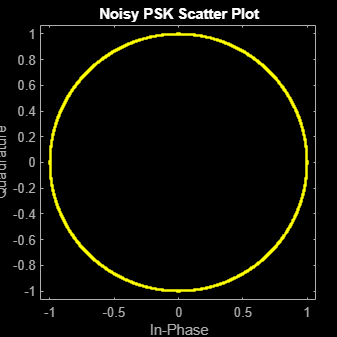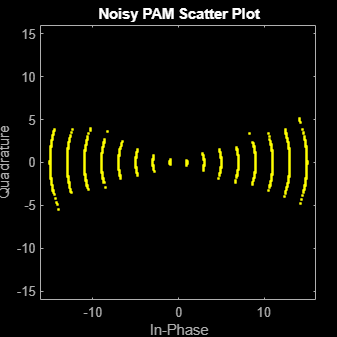Documentation

# pskdemod

Phase shift keying demodulation

## Syntax

``z = pskdemod(y,M)``
``z = pskdemod(y,M,ini_phase)``
``z = pskdemod(y,M,ini_phase,symorder)``

## Description

example

````z = pskdemod(y,M)` demodulates the complex envelope, `y`, of a PSK-modulated signal having modulation order `M`.```

example

````z = pskdemod(y,M,ini_phase)` specifies the initial phase of the PSK-modulated signal.```
````z = pskdemod(y,M,ini_phase,symorder)` specifies the symbol order of the PSK-modulated signal.```

## Examples

collapse all

Compare PSK and PAM modulation schemes to demonstrate that PSK is more sensitive to phase noise. This is the expected result because the PSK constellation is circular while the PAM constellation is linear.

Specify the number of symbols and the modulation order parameters. Generate random data symbols.

```len = 10000; M = 16; msg = randi([0 M-1],len,1);```

Modulate `msg` using both PSK and PAM to compare the two methods.

```txpsk = pskmod(msg,M); txpam = pammod(msg,M);```

Perturb the phase of the modulated signals by applying a random phase rotation.

```phasenoise = randn(len,1)*.015; rxpsk = txpsk.*exp(2i*pi*phasenoise); rxpam = txpam.*exp(2i*pi*phasenoise);```

Create scatter plots of the received signals.

```scatterplot(rxpsk); title('Noisy PSK Scatter Plot')``````scatterplot(rxpam); title('Noisy PAM Scatter Plot')``````recovpsk = pskdemod(rxpsk,M); recovpam = pamdemod(rxpam,M);```

Compute the number of symbol errors for each modulation scheme. The PSK signal experiences a much greater number of symbol errors.

```numerrs_psk = symerr(msg,recovpsk); numerrs_pam = symerr(msg,recovpam); [numerrs_psk numerrs_pam]```
```ans = 1×2 343 1 ```

Generate random symbols.

`dataIn = randi([0 3],1000,1);`

QPSK modulate the data.

`txSig = pskmod(dataIn,4,pi/4);`

Pass the signal through an AWGN channel.

`rxSig = awgn(txSig,10);`

Demodulate the received signal and compute the number of symbol errors.

```dataOut = pskdemod(rxSig,4,pi/4); numErrs = symerr(dataIn,dataOut)```
```numErrs = 2 ```

## Input Arguments

collapse all

PSK-modulated input signal, specified as a real or complex vector or matrix. If `y` is a matrix, the function processes the columns independently.

Data Types: `single` | `double`
Complex Number Support: Yes

Modulation order, specified as an integer power of two.

Example: `2` | `4` | `16`

Data Types: `double` | `single`

Initial phase of the PSK modulation, specified in radians as a real scalar.

If `ini_phase` is empty, then `pskdemod` uses an initial phase of 0.

Example: `pi/4`

Data Types: `double` | `single`

Symbol order, specified as `'bin'` or `'gray'`. This argument specifies how the function assigns binary vectors to corresponding integers.

• If `symorder` is `'bin'`, the function uses a natural binary-coded ordering.

• If `symorder` is `'gray'`, the function uses a Gray-coded ordering.

Data Types: `char`

## Output Arguments

collapse all

PSK-demodulated output signal, returned as a vector or matrix having the same number of columns as input signal `y`.

Data Types: `double` | `single`